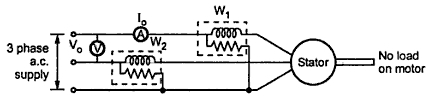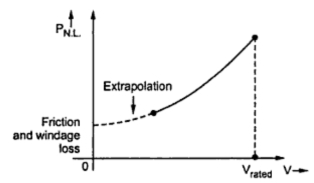In this test, the motor is made to run without any load i.e. no load condition. The speed of the motor is very close to the synchronous speed but less than the synchronous speed. The rated voltage is applied to the stator. The input line current and total in put power is measured. The two wattmeter method is used to measure the total input power. The circuit diagram for the test is shown in the Fig. 1.Fig. 1 No load test

As the motor is on no load, the power factor is very low which is less than 0.5 and one of the two wattmeters reads negative. It is necessary to reverse the current coil or pressure coil connections of such a wattmeter to get the positive reading. This reading must be taken negative for the further calculations.
The total power input Wo is the algebraic sum of the two wattmeter readings. The observation table is,

The calculations are,
Wo = √3VIo cosΦo
This is no load power factor.
Thus we are now in a position to obtain magnitude and phase angle of no load current Io, which is required for the circle diagram.
From the knowledge of Io and Φo, the parameters of the equivalent circuit can be obtained as,
Ic = Io cosΦo = Active component of no load current
Im = Io sinΦo = Magnetising component of no load current
Ro = Vo (per phase) / Ic (per phase) = No load branch resistance
Xo = Vo (per phase)/ Im (per phase) = No load branch resistance
The power input Wo consists of following losses,
1. Stator copper loss i.e. 3 Io2 R12where  I is no load per phase current and R1 is stator resistance per phase.
2. Stator core loss i.e. iron loss.
3. Friction and windage loss.
The no load rotor current is very small and hence rotor copper loss is negligibly small. The rotor frequency is s times supply frequency and on no load it is very small. Rotor iron losses are proportional to this frequency and hence are negligibly small.
Key Point : Under no load condition, I is also very small and in many practical cases it is also neglected.
Thus Wo consists of stator iron loss and friction and windage loss which are consists for all load condictions. Hence Wo is said to give fixed losses of the motor.
...                  Wo = No load power inputFig. 3